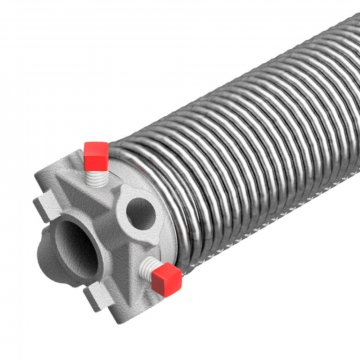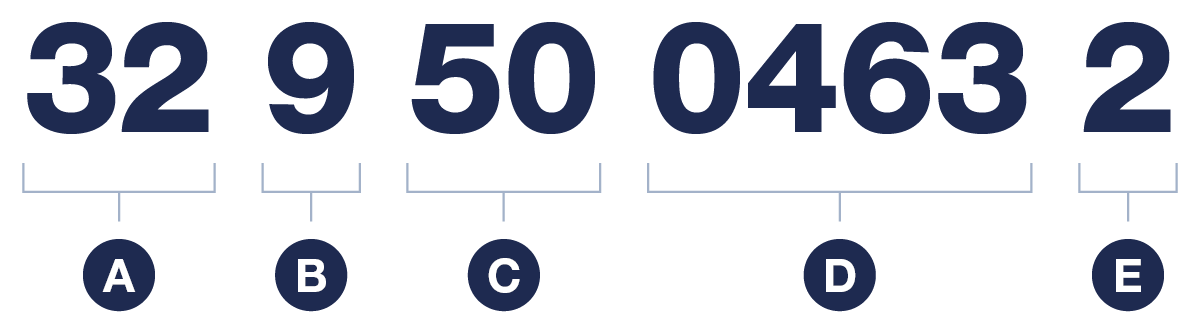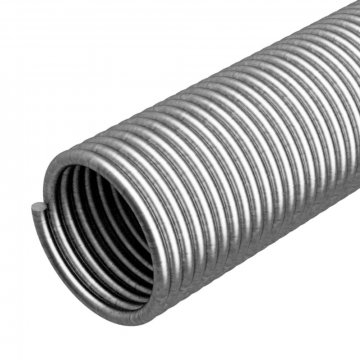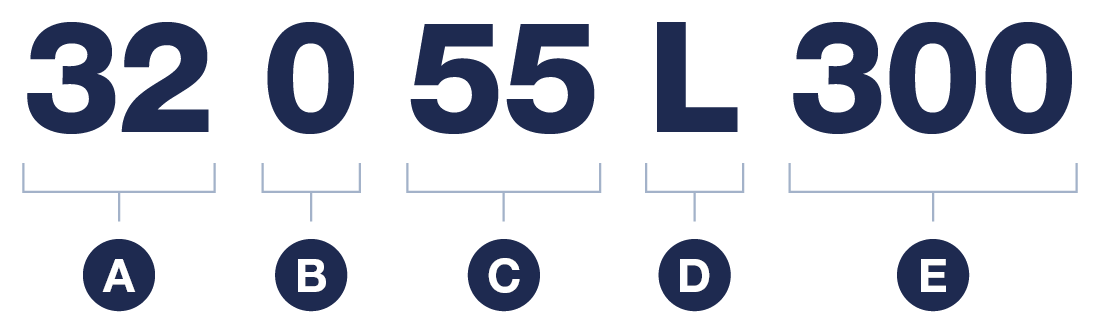# Spring calculators Support# Preassembled torsion spring sets

The article numbers of our preassembled torsion spring sets are easy to identify using the overview below. This way, you can save time identifying what spring you are dealing with.A Inner diameter 32 = 50,8mm 33 = 66,68mm B Finish 9 = Galvanized C Wire diameter 50 = 5,0mm 53 = 5,3mm 55 = 5,5mm 60 = 6,0mm 65 = 6,5mm 70 = 7,0mm D Length mm E Unit 2 = Pair

Please note: not all combinations are possible.# Torsion springs

The article numbers of our torsion springs are easy to identify using the overview below. This way, you can save time identifying what spring you are dealing with.A Inner diameter 32 = 50,8mm 33 = 66,68mm 34 = 95,25mm 36 = 152,4mm B Finish 0 = Black 7 = Shot blasted 9 = Galvanized C Wire diameter 45 = 4,5mm 50 = 5,0mm 55 = 5,5mm 60 = 6,0mm 65 = 6,5mm 70 = 7,0mm 75 = 7,5mm 80 = 8,0mm 85 = 8,5mm 90 = 9,0mm 95 = 9,5mm 10 = 10,0mm 05 = 10,5mm 11 = 11mm 15 = 11,5mm 12 = 12mm D Direction L = Left (Red) R = Right (Black / blue) E Length cm

Please note: not all combinations are possible.